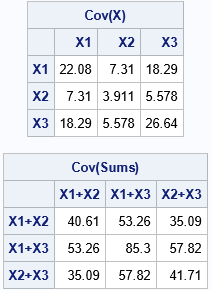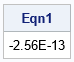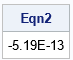Undergraduate textbooks on probability and statistics typically prove theorems that show how the variance of a sum of random variables is related to the variance of the original variables and the covariance between them. For example, the Wikipedia article on Variance contains an equation for the sum of two random variables, X and Y:
$\operatorname {Var} (X+Y)=\operatorname {Var} (X)+\operatorname {Var} (Y)+2\,\operatorname {Cov} (X,Y)$

A SAS programmer wondered whether equations like this are also true for vectors of data. In other words, if X and Y are columns in a data set, do the sample variance and covariance statistics satisfy the same equation? How can you use SAS to verify (or disprove) the equation for a sample of data? This article shows how to use SAS to verify that the equation (and another similar equation) is valid for vectors of data.

It is possible to verify the equation by using PROC CORR and the DATA step, but it is much simpler to use SAS IML software because the IML language enables you to directly access cells of a variance-covariance matrix.

### Create the sum of columns

Let's start by choosing some data to analyze. The following DATA step renames some variables in the Sashelp.Iris data set to X1, X2, and X3. The program also creates the variables Y12 = X1 + X2, Y13 = X1 + X3, and Y23 = X2 + X3. The program then reads the data into SAS IML matrices and computes the variance-covariance matrix for the original variables (X) and their pairwise sums (Y):

/* verify variance of a sum (or linear combination) of variables */ data Want; set sashelp.iris(where=(Species="Versicolor")); X1 = PetalLength; X2 = PetalWidth; X3 = SepalLength; Y12 = X1 + X2; Y13 = X1 + X3; Y23 = X2 + X3; keep X1-X3 Y12 Y13 Y23; run;   proc iml; use Want; read all var {'X1' 'X2' 'X3'} into X[c=XNames]; read all var {'Y12' 'Y13' 'Y23'} into Y; close; YNames = {'X1+X2' 'X1+X3' 'X2+X3'};   SX = cov(X); SY = cov(Y); print SX[c=XNames r=XNames F=best5. L='Cov(X)'], SY[c=YNames r=YNames F=best5. L='Cov(Sums)'];### The variance of a sum

Let's use this information to verify the identity
$\operatorname {Var} (X1+X2)=\operatorname {Var} (X1)+\operatorname {Var} (X2)+2\,\operatorname {Cov} (X1,X2)$

From the displayed (rounded) values of the covariance matrices, you can mentally calculate that the equation could be true. The variances of the original variables are along the diagonal of the first matrix, and the covariances are the off-diagonal elements. The variance of the sum is the [1,1] cell of the second matrix. A little mental arithmetic indicates that 40.6 ≈ 22 + 4 + 2*7.

The following SAS IML statements extract the relevant values from cells in the variance-covariance matrices. The program then subtracts the right side of the equation from the left side. If the difference is 0, then the equation is verified:

/* Confirm that the sample variance of (x1+x2) satisfies Var(x1 +x2) = Var(x1) + Var(x2) + 2*Cov(x1, x2) See https://en.wikipedia.org/wiki/Variance#Propagation */ VarY12= SY[1,1]; VarX1 = SX[1,1]; VarX2 = SX[2,2]; Cov12 = SX[1,2]; Eqn1 = VarY12 - (VarX1 + VarX2 + 2*Cov12); print Eqn1;Success! The left and right sides of the equation are equal to numerical precision. This validates the equation for the data we are using. Notice that this same technique could be used to analyze the variance of a general linear combination of other variables.

### The covariance of sums

Let's verify one more equation. The Wikipedia article about Covariance states the following formula for four random variables, X, Y, Z, and W:
$\operatorname {Cov} (X+Y,Z+W)=\operatorname {Cov} (X,Z)+\operatorname {Cov} (X,W)+\operatorname {Cov} (Y,Z)+\operatorname {Cov} (Y,W)$

Since our example data only has three variables, let's simplify the equation by setting W=X. Then (changing variable names) the equation we want to verify is $\operatorname {Cov} (X1+X2,X1+X3)=\operatorname {Cov} (X1,X3)+\operatorname {Cov} (X1,X1)+\operatorname {Cov} (X2,X3)+\operatorname {Cov} (X1,X2)$

The following SAS IML statements extract the relevant cells from the covariance matrices. The program subtracts the right side of the equation from the left side and prints the difference.

/* Confirm that the sample covariance of (x1+x2) and (x1 + x3) satisfies Cov(x1+x2, x1+x3) = Cov(x1, x1) + Cov(x1, x2) + Cov(x1, x3) + Cov(x2, x3) See https://en.wikipedia.org/wiki/Covariance#Covariance_of_linear_combinations */ CovY12_Y13 = SY[1,2]; Cov11 = SX[1,1]; /* = Var(X1) */ Cov12 = SX[1,2]; Cov13 = SX[1,3]; Cov23 = SX[2,3]; Eqn2 = CovY12_Y13 - (Cov11 + Cov12 + Cov13 + Cov23); print Eqn2;Once again, the difference is essentially zero, which validates the equation.

### Summary

A SAS programmer asked whether she could use SAS to verify certain equations. She wanted to verify that certain formulas for the variance and covariance of random variables are also true for sample statistics for empirical data. This article shows how to use SAS IML software to test certain equations that relate the variance and covariance of sums to the variances and covariances of a set of data variables.

The post The variance of the sums of variables appeared first on The DO Loop.